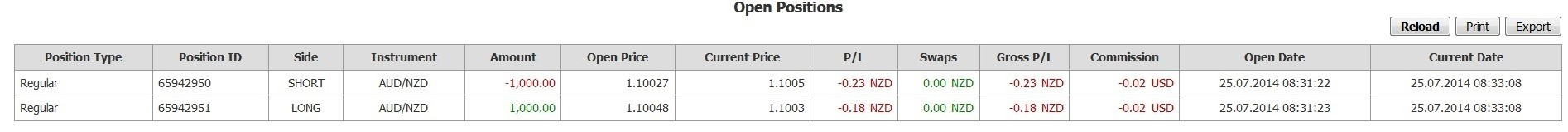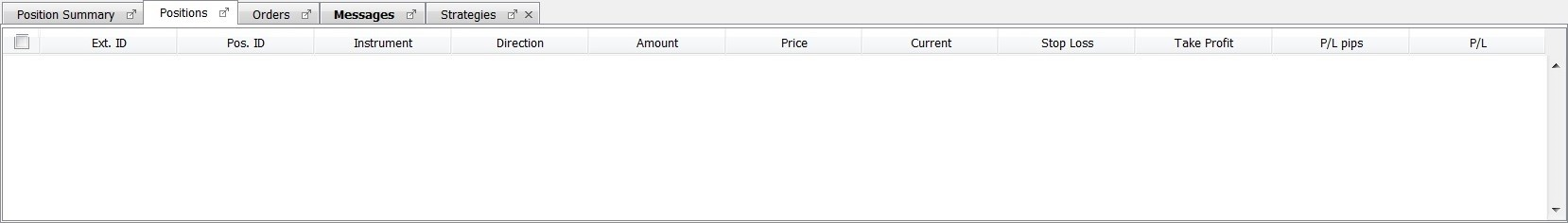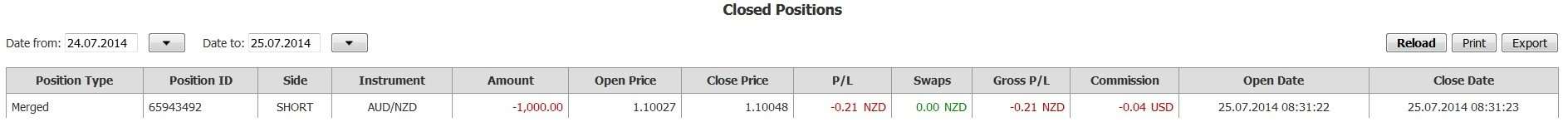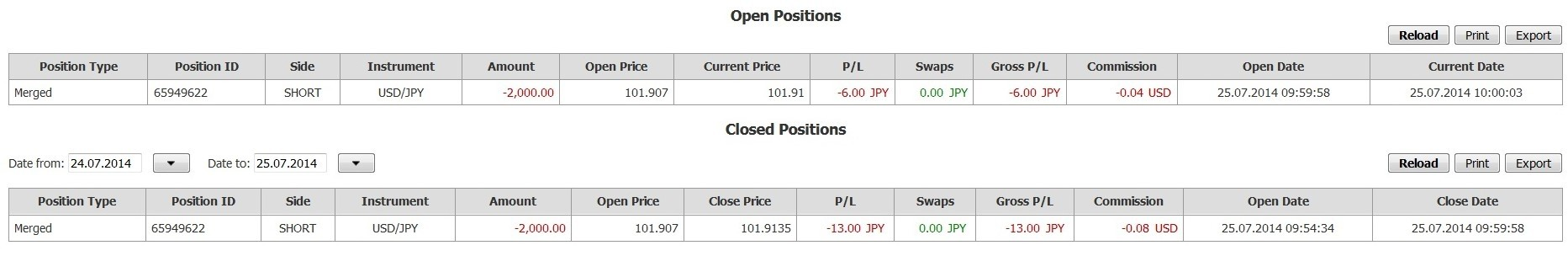# Merge

The trading platforms allow the traders to take and maintain hedged exposures, that is, both long and short positions in a given instrument.

The merge operation aims to deal efficiently with hedged exposures. Merge is the operation that offset several positions between them without any market transaction hence implying NO COMMISSION nor spread costs. The merge operation can be performed on hedged positions as well as on no hedged exposures provided there is more than one position in a given instrument.

The merge operation has multiple purposes. It is used either to reduce the number of positions in a given instrument for either clarity or exposure management purposes, or it can be performed to reduce several positions into the net exposure position.

`````` A maximum of 100 individual positions /actually open or potential (pending orders) can be open in the DEMO environment, while the maximum is 200 in the LIVE environment. Orders linked to a position (take-profit and stop-loss orders) are not taken into account in this maximum. Should the number of open positions/pending orders reach the maximum, the trader is prevented for opening new positions, or enter new pending orders.
A message is dispatched upon such event warning the trader in the message log, and in the activity log. Merging open positions in part or in full and/or cancellation of pending orders, will decrease the number of open positions/pending orders, therefore enabling the trader to open new individual positions, and/or enter new further pending orders.``````
``Merge is an optional operation. It is the entire responsibility of the trader to use or not the merge operation. There is no situation where the merge operation would be performed automatically except when the minimum account equity procedure is enforced. Therefore, traders must carefully monitor their hedged exposures. Clients must be bear in mind that in some situations, the merge process is an economical solution by contrast with the close order, basically when hedged exposures are to be considered. Even in that case however, it remains the entire responsibility of the trader to merge or close eventually. Dukascopy Bank SA shall bear no responsibility in situations where the clients suffer commission and/or spread costs due to the decision to close instead of merge. With this respect, clients are encouraged to read thoroughly the sections about the margin cut trade processing and other sections dealing with partial, or full hedged exposures.``

## How to merge positions?

At the bottom of the window, on the position Tab, select the positions you want to merge by ticking them on the first column. Them right click on one of the positions and choose “Merge selected”. DONE!

## How to calculate the new price and amount of the unique position created?

### Positions in same direction

To calculate the price of the future position, multiply the price of each position by the amount of the position and sum the results. Than you divide this number by the total amount of the positions. If the two positions have the same amount, just add the prices and divide the result by 2.

The amount of the new position will be the addition of the amounts.

### Positions in different direction

As shown below, merging two offsetting transactions (of equal amount) will result in closing of both positions as one.

This is how 2 positions look in the platform before merge:This is how 2 positions look like in the position report before merge:This is how the same 2 positions look like in the platform after merge:And this is how the same 2 positions look like in the position report after merge:Please note, that the Closed position Side is shown as “SHORT“, since the first by time opened position that was involved in the merge was “SHORT”. Merging several positions of different amounts will result in closing of the offsetting parts and opening of a new position. Position ID for the closed part and open part will be the same.

#### Example

6 positions are open on USD/JPY:After the merge:1. Total SHORT amount is 4000 USD, Total LONG amount is 2000 USD. Resulting amount for open position = 4000 – 2000 = 2000 USD short. Offsetting parts are 2000 USD short and 2000 USD long, so closed position is 2000 USD short.

2. The Opening price of the closed part is going to be calculated as following:

1. For the first by time opened position, amount is multiplied by Open Price

2. The same is done for all positions in the same direction

3. The sum of 2.1 and 2.2 is taken and divided by the sum of all amounts of 2.1 and 2.2

As in our example,
1000 x 101.906 = 101906
1000 x 101.907 = 101907
1000 x 101.907 = 101907
1000 x 101.908 = 101908

(101906+101907+101907+101908) / (1000+1000+1000+1000) = 101.907

3. The Current Price ( Close price ) of the closed part is going to be calculated as following:

1. For all positions that are opposite by direction to the first by time opened position, amount is multiplied by Open Price

2. The sum of 3.1 is taken and divided by the sum of all amounts of 3.1

As in our example,
1000 x 101.912 = 101912
1000 x 101.915 = 101915

(101912+101915) / (1000+1000) = 101.9135

4. The Opening price of the open part is going to be calculated as following:

1. If the closed part was in the same side, as the remaining open part, open price is going to be calculated as sum of all positions in that side multiplied by their corresponding amounts, and divided by the sum of their amounts

2. If the closed part was in the opposite side, as the remaining open part, open price is going to be the same, as the “ Current Price “ for the close part

As in our example, the close part is “SHORT” and the remaining part is “SHORT“, we will use the 4.1 formula to calculate open price,

1000 x 101.906 = 101906
1000 x 101.907 = 101907
1000 x 101.907 = 101907
1000 x 101.908 = 101908

(101906+101907+101907+101908) / (1000+1000+1000+1000) = 101.907

5. The Current Price of the open part is going to be the same, as the current market price of the position currency pair.

The information on this web site is provided only as general information, which may be incomplete or outdated. Click here for full disclaimer.